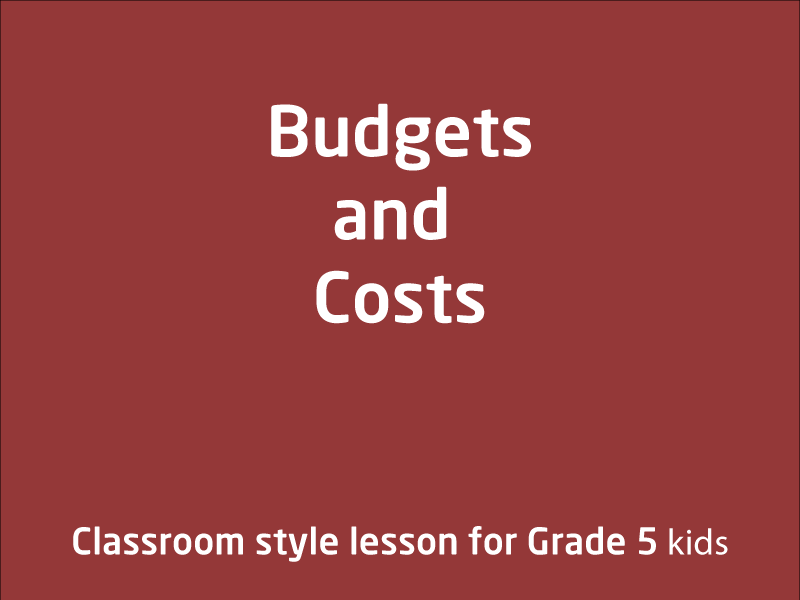# Free video lessons for Foundation to Grade 8 students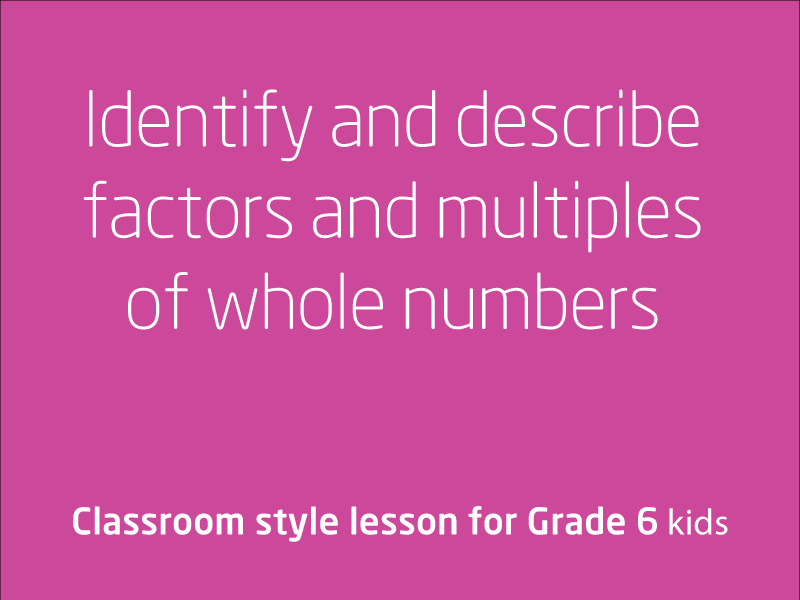### Identify and describe factors and multiples of whole numbers

This lesson starts with a bit of re-introduction to decimal place values before jumping into an explanation on multiples and factors. This is starters lesson but handy for students...

1 videos
Intermediate difficulty
0 0 0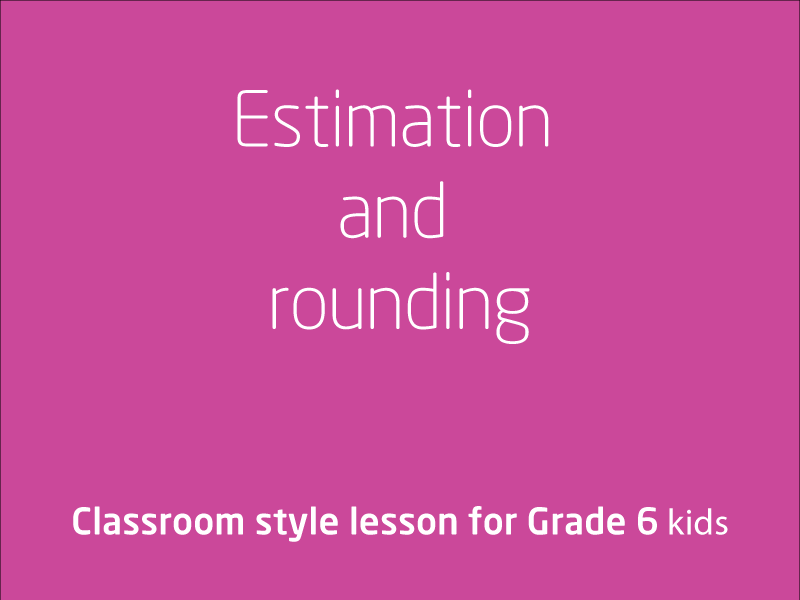### Estimation and rounding

This lesson is all about estimation and rounding. Your coach will use estimation and rounding to check the reasonableness of answers to calculations.Elaborationsrecognisi...

1 videos
Intermediate difficulty
0 0 0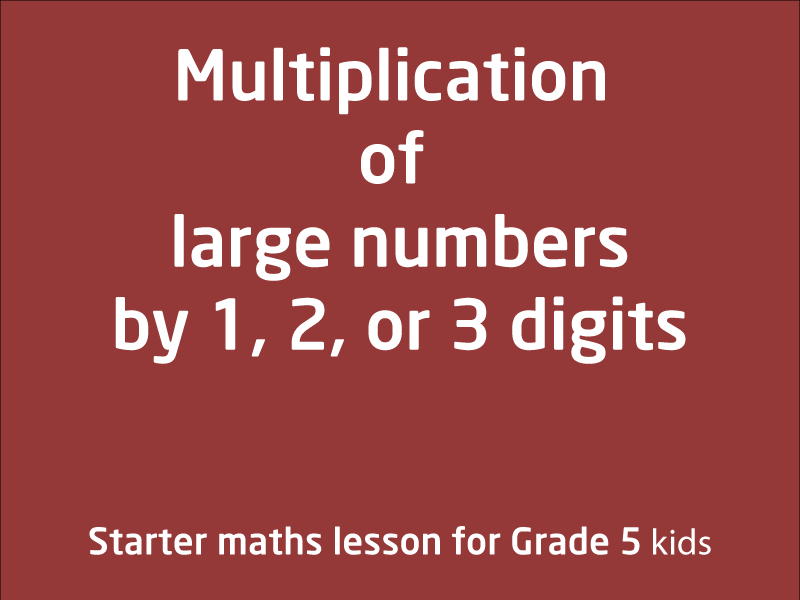### Multiplication of large numbers

In this video lesson, your coach will show you some techniques on how to solve problems involving multiplication of large numbers by one- two-or three-digit numbers using...

1 videos
Intermediate difficulty
0 0 0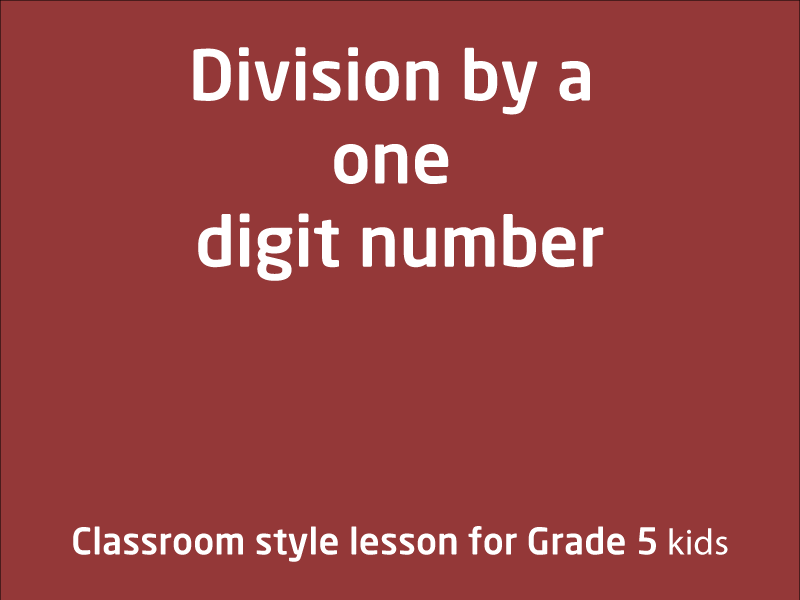### Division by a one digit number, including those that result in a remainder

In this video lesson, you coach will teach you techniques that will make it simple for you to divide any number with single digit numbers.Elaborationsusing the fact that equivalent...

1 videos
Intermediate difficulty
0 0 0### Efficient strategies for solving Four operation problems

This is a generic lesson where we learn about some strategies to deal with addition, subtraction, multiplication and division. This lesson is suitable for Grade 4,5 and 6 students ...

1 videos
Intermediate difficulty
0 0 0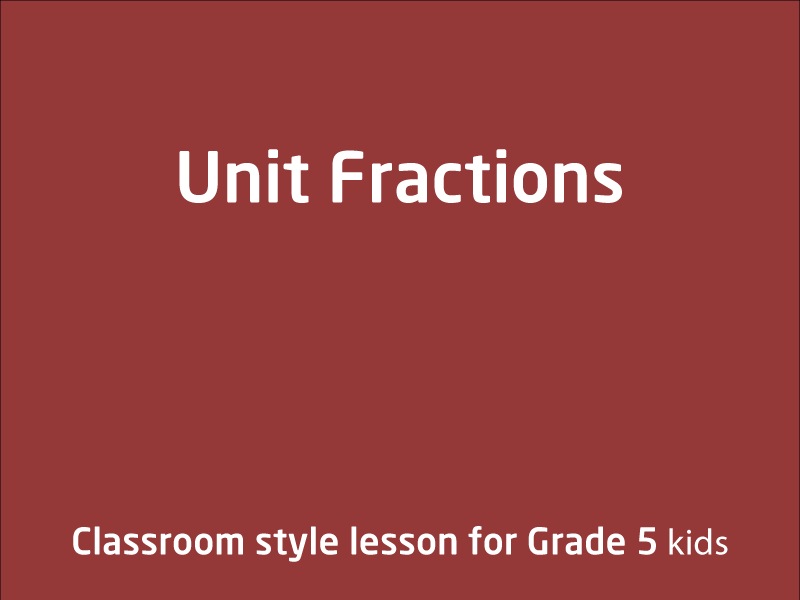### Unit Fractions

In this video lesson, we will be comparing and ordering common unit fractions and locate and represent them on a number lineElaborationsrecognising the connection between the ...

1 videos
Intermediate difficulty
0 0 0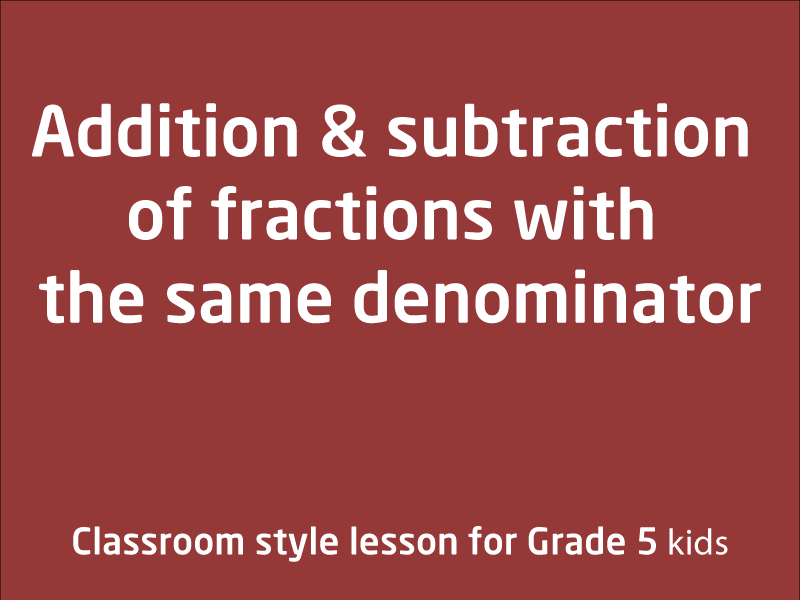### Addition and subtraction of fractions with the same denominator

In this video lesson you will learn techniques on how to do addition and subtraction of fractions with the same denominator.Elaborationsmodelling and solving addition and...

1 videos
Intermediate difficulty
0 0 0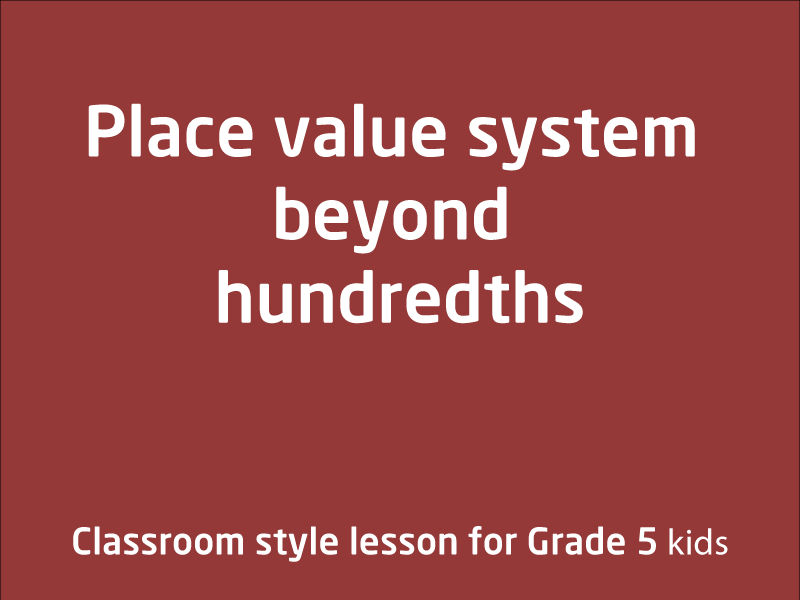### Place value system beyond hundredths

In this video lesson, your tutor will show you how  the place value system can be extended beyond hundredths. There are several examples provided to help you understand t...

1 videos
Intermediate difficulty
0 0 0### Compare, order and represent decimals

In this video lesson, you shall look forward to learn more about decimals, and how to compare them. ElaborationsCovers locating decimals on a number line...

1 videos
Intermediate difficulty